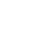# Exchange Rate Determination Models

Determination of the exchange rate is as simple as the determination of price of any commodity or product or service. Only thing, here the commodity itself is one currency, so price of one currency in terms of another is required. But the caveat is determination of price of any commodity/product/service is not that simple. The determinants of the exchange rate are too many to consider. Yet certain macro variables would capture the same.

Flow models and asset models are used in exchange rate determination. These are explained below:

### 1. Flow Model

The flow model of exchange rate determination simply is based on demand and supply of Forex. Demand for foreign exchange takes place whenever a country imports goods and services, people of a country undertake visits to other countries, citizens of a country remit money abroad and whatever purpose, business units set up foreign subsidiaries and so on. In all these cases the nation concerned buys relevant and required foreign exchange, in exchange of its own currency, or draws from foreign exchange reserves built. So the demand side includes importers, citizens undertaking outward travel, remittances against foreign services obtained, outward foreign debt servicing, export of capital for overseas investment, buying of Forex by monetary authority as an intervention strategy, etc.

On the other hand, when a country exports goods and services to another country, when people of other countries visit the country, when citizens of the country settled abroad remit money homewards, when foreign citizens, firms and institutions invest in the country and when the country or its business community raises funds from abroad, the country’s currency is brought by others, giving foreign exchange, in exchange, inflow of foreign exchange takes place. So the supply side includes exporters, foreign citizens undertaking inward travel, remittances against foreign services provided, inward foreign debt servicing, inflow of capital in the form of foreign direct investment, portfolio investment, release of Forex from Forex reserve by the monetary authority, etc.

### 2. Current Account Monetary Model

The model assumes that: There is only one asset that is money. Domestic money is held by residents of the country and foreign money by foreigners only. Purchasing Power Parity theory holds good. There is stable demand for money in each country. Demand for money depends on real income and nominal interest rate. Foreign real income and nominal interest rate are external variables not influenced by domestic factors. Fully flexible exchange rate system is followed keeping the exchange rate in continuous equilibrium. With these assumptions the equilibrium exchange rate in ‘direct quote form:

‘S’= k + Ãœ (m-m) — Ã¢ (y-y) + Ã¨ (i-i)

Where ‘S’- the exchange rate. A higher ‘S’ means foreign currency appreciates and lower ‘S’ means it depreciates.

• k is a constant,
• Ãœ is regression coefficient attached to (mh-mf) ,mh is domestic money supply, mf foreign money supply,
• Ã¢ is regression coefficient attached to (yh-yf), h is real domestic income ,yf is real foreign income ,
• Ã¨ is regression coefficient attached to (ih-if), is nominal domestic interest rate, h if is nominal foreign interest rate.

f (mh-mf) > 0, domestic money supply exceeds foreign money supply and this leads to increase in S , that means domestic currency depreciates or foreign currency appreciates, because the regression is positive coefficient. If (yh-yf) > 0, domestic real income exceeds foreign real income and this leads to decrease in S , that means domestic currency appreciates or foreign currency depreciates, because the regression is a negative coefficient.
If (ih-if)> 0, domestic nominal interest rate exceeds foreign nominal interest rate and this leads to increase in S , that means domestic currency depreciates or foreign currency appreciates, because the regression is positive coefficient.

### 3. Capital Account Monetary Model

The capital account model assumes that: Purchasing Power Parity theory holds good. There is stable demand for money in each country. Demand for money depends on real income and nominal interest rate. Foreign real income and nominal interest rate are external variables not influenced by domestic factors. Uncovered interest parity theory holds good and Fisher open condition exists. Expected change in exchange rate depends on perceived departures from long-term equilibrium exchange rate. With these assumptions the equilibrium exchange rate in ‘direct quote form:

‘S’= k + Ãœ (M-M) — Ã¢(Y-Y) + Ã¨ (I-I) + Ã« ( Ã¯-Ã¯)

Where ‘S’- the exchange rate. A higher S means foreign currency appreciates and lower S means it depreciates.

• k is a constant,
• Ãœ is regression coefficient attached to (mh-mf), mh is domestic money supply, mf foreign money supply
• Ã¢ is regression coefficient attached to (yh-yf), yh is real domestic income, yf is real foreign income
• Ã¨ is regression coefficient attached to (ih-if), ih nominal domestic interest rate, if is nominal foreign interest rate
• Ã« is regression coefficient attached to ( Ã¯h-Ã¯f), Ã¯is domestic inflation, Ã¯f foreign inflation.

If (Mh-Mf) > 0, long-term domestic money supply exceeds long-term foreign money supply and this leads to increase in S , that means domestic currency depreciates or foreign currency appreciates, because the regression is positive coefficient.
If (yh-yf) > 0, long-term domestic real income exceeds long-term foreign real income and this leads to decrease in S , that means domestic currency appreciates or foreign currency depreciates, because the regression is a negative coefficient.
If (ih-if)> 0, long-term domestic nominal interest rate exceeds long-term foreign nominal interest rate and this leads to increase in S , that means domestic currency depreciates or foreign currency appreciates, because the regression is positive coefficient.
If (Ã¯h-Ã¯f)> 0, long-term domestic inflation rate exceeds long-term foreign inflation rate and this leads to increase in S , that means domestic currency depreciates or foreign currency appreciates, because the regression is positive coefficient.

## One thought on “Exchange Rate Determination Models”

1.Priyam says: